# The Fourier Transform

In the previous three lessons, we discussed the Fourier Series, which is for periodic signals. This lesson will cover the Fourier Transform which can be used to analyze aperiodic signals. (Later on, we'll see how we can also use it for periodic signals.) The Fourier Transform is another method for representing signals and systems in the frequency domain.

### Definition of the Fourier Transform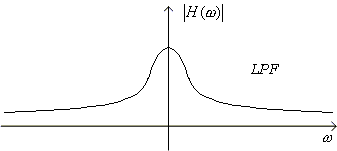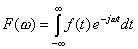is the continuous time Fourier transform of f(t).

It is an extension of the Fourier Series. The Fourier transformation creates F(ω) in the FREQUENCY domain. We will see that F(ω) can be seen as a "continuous coefficient" of a Fourier Series if we let the period of a periodic function go to infinity so that the resulting function becomes aperiodic in the limit.

Let fp(t) be a periodic function. Therefore, we can express it with a Fourier series: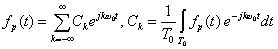As usual,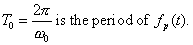Assuming that fp(t) is a periodic rectangular pulse train, let's plot its magnitude spectrum Ck vs. ω=kω0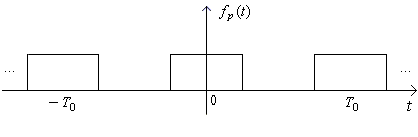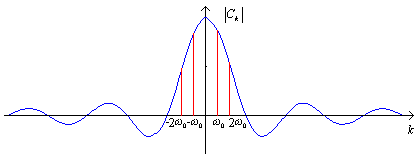As shown, each Ck is the frequency component of fp(t) at the frequency ω = k ω0.

Now, if we let T0, the period of fp(t), go to infinity (meaning that fp(t) becomes aperiodic), then the fundamental frequency ω0 will go to zero. Then the lines in the plot will get closer and closer together and merge into a continuous spectrum.

As ω0 0, the distance between lines goes to 0, so let's write Δω for ω0 in our Fourier Series representation of fp(t):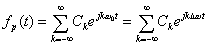Next, we can replace Ck in our Fourier Series representation of fp(t) by using the formula we derived in Lesson 13 for the Fourier Series coefficients: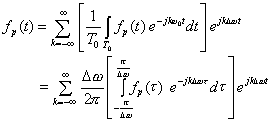Next,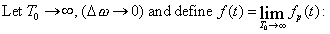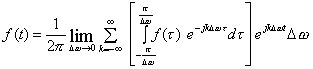As Δω → 0, we write it as and the sum becomes an integral (and kΔω approaches ω as T0 → ∞ where ω is a continuous variable).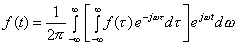Let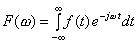be the Fourier Transform of f(t).

So F(ω) replaces the Ck as Δω → 0 and is a continuous function of ω.

Finally, we have derived our Fourier transform pair: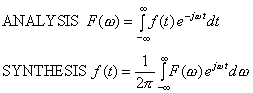Notice the similarity between the two formulas except for the sign change in the exponent and the multiplicative factor in front of the synthesis formula.

Because the Fourier Transform is an integral over an infinite range, we must consider whether or not the integral converges. Sufficient conditions for the existence of the Fourier Transform are the Dirichlet conditions. That is, the Fourier Transform exists if:

1.   On any finite interval

(a)  f(t) is bounded
(b)  f(t) has a finite number of minima and maxima
(c)  f(t) has a finite number of discontinuities

2.    f(t) is absolutely integrable, that is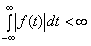This can be seen because we know that |e-jωt| = 1: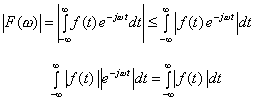Therefore, if f(t) is absolutely integrable, then its Fourier Transform exists.

3.    Basically, if you can generate a signal in a laboratory, since it has finite energy, it will have a Fourier Transform.

Now, let's compute our first Fourier Transforms:

Example 1

Example 2

Example 3

Example 4

Example 5

Example 6